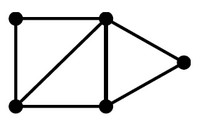adjacent is a graph operator that tests if there is an edge between two vertices;

The two vertices have the following binary relation, if the test is:

• false: is not adjacent to

A synonym for adjacent is connected

Discover MoreGraph - Data Structure (Physical Representation)

A graph is represented generally in a physical data structure The graph is composed of two set. a set of vertices (node) Node a b c and set of egde represented for a : directed graph...Graph - Degree

In a graph, the degree of a vertex (node) is the number of edges that touch the node (vertex) (ie the number of adjacent edges) degree is also known as valency The original 'six degrees of separation'...Graph - Operator (Operations)

on a graph Operations Description degree(G, x) the number of adjacent (connected) edge for the node x (ie degree) adjacent(G, x, y) adjacent tests whether there is an edge from the vertex x...Graph - Relationship

in Graph neighbors are the node that are adjacent (directly connected) to another node.Logical Data Modeling - Binary Relation

A binary relation is a relationship between two elements that is implemented via a binary function. Binary relations are used in many branches of mathematics to model concepts like: order relation...